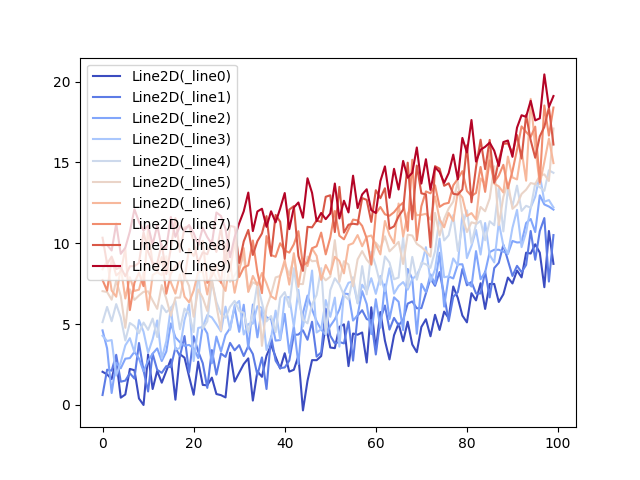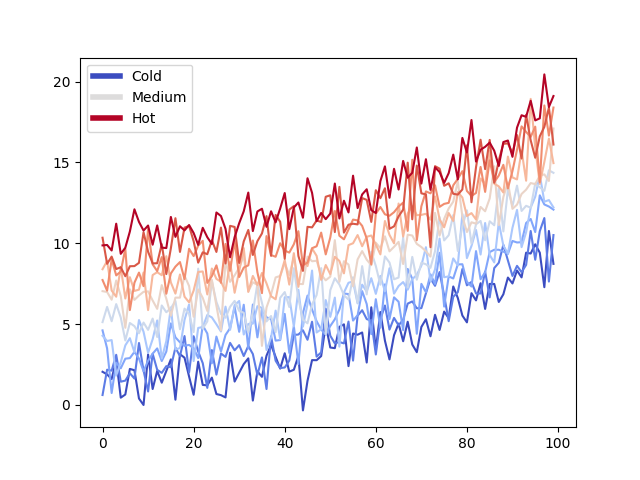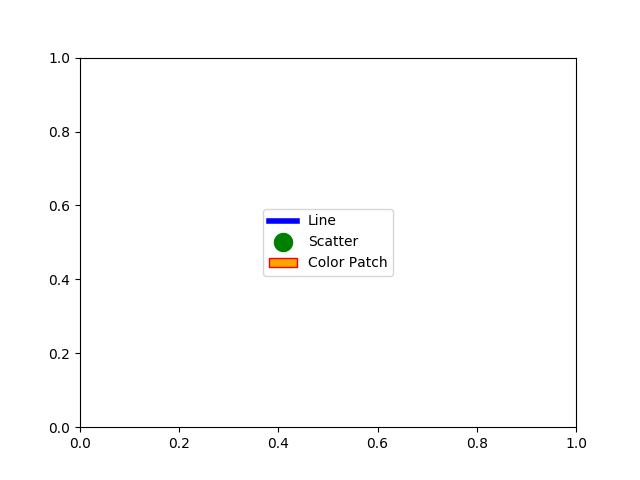You are reading an old version of the documentation (v3.0.0). For the latest version see https://matplotlib.org/stable/gallery/text_labels_and_annotations/custom_legends.html

# Composing Custom Legends¶

Composing custom legends piece-by-piece.

Note

For more information on creating and customizing legends, see the following pages:

Sometimes you don't want a legend that is explicitly tied to data that you have plotted. For example, say you have plotted 10 lines, but don't want a legend item to show up for each one. If you simply plot the lines and call `ax.legend()`, you will get the following:

```# sphinx_gallery_thumbnail_number = 2
from matplotlib import rcParams, cycler
import matplotlib.pyplot as plt
import numpy as np

# Fixing random state for reproducibility
np.random.seed(19680801)

N = 10
data = [np.logspace(0, 1, 100) + np.random.randn(100) + ii for ii in range(N)]
data = np.array(data).T
cmap = plt.cm.coolwarm
rcParams['axes.prop_cycle'] = cycler(color=cmap(np.linspace(0, 1, N)))

fig, ax = plt.subplots()
lines = ax.plot(data)
ax.legend(lines)
```Note that one legend item per line was created. In this case, we can compose a legend using Matplotlib objects that aren't explicitly tied to the data that was plotted. For example:

```from matplotlib.lines import Line2D
custom_lines = [Line2D(, , color=cmap(0.), lw=4),
Line2D(, , color=cmap(.5), lw=4),
Line2D(, , color=cmap(1.), lw=4)]

fig, ax = plt.subplots()
lines = ax.plot(data)
ax.legend(custom_lines, ['Cold', 'Medium', 'Hot'])
```There are many other Matplotlib objects that can be used in this way. In the code below we've listed a few common ones.

```from matplotlib.patches import Patch
from matplotlib.lines import Line2D

legend_elements = [Line2D(, , color='b', lw=4, label='Line'),
Line2D(, , marker='o', color='w', label='Scatter',
markerfacecolor='g', markersize=15),
Patch(facecolor='orange', edgecolor='r',
label='Color Patch')]

# Create the figure
fig, ax = plt.subplots()
ax.legend(handles=legend_elements, loc='center')

plt.show()
```Keywords: matplotlib code example, codex, python plot, pyplot Gallery generated by Sphinx-Gallery